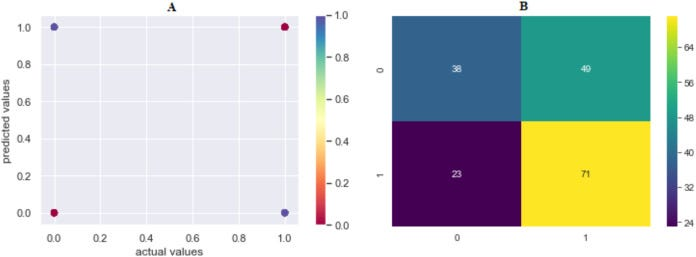# ⛓️Cyber Crime cases where they talk about “confusion matrix”

This problem can be tackled by identifying an attack and the perpetrator of such attack, using actual data. The data include the type of crime, gender of perpetrator, damage and methods of attack. The data can be acquired from the applications of the persons who were exposed to cyber-attacks to the forensic units.

The Logistic Regression was the leading method in detecting attackers with an accuracy rate of 65.42%.

In other method, we predicted whether the perpetrators could be identified by comparing their characteristics.

# But ,(A) Model 2 comparison values (results are become more red as they approach the actual values and more purple as they move away); (B) confusion matrix of predicted values.

Where 0 is “Perpetrator Known”, 1 is “Perpetrator Unknown”.

Example:💡

In this confusion matrix, there are 19 total predictions made. 14 are correct and 5 are wrong.

The False Negative cell, number 3, means that the model predicted a negative, and the actual was a positive.

The False Positive cell, number 2, means that the model predicted a positive, but the actual was a negative.

The false positive means little to the direction a person chooses at this point. But, if you added some stakes to the choice, like choosing right led to a huge reward, and falsely choosing it meant certain death, then now there are stakes on the decision, and a false negative could be very costly. We would only want the model to make the decision if it were 100% certain that was the choice to make.

The confusion matrix gives you a lot of information, but sometimes you may prefer a more concise metric.

Precision:Precision measures how good our model is when the prediction is positive. It is the ratio of correct positive predictions to all positive predictions
precision = (TP) / (TP+FP)

TP is the number of true positives, and FP is the number of false positives.
A trivial way to have perfect precision is to make one single positive prediction and ensure it is correct (precision = 1/1 = 100%). This would not be very useful since the classifier would ignore all but one positive instance.

Recall:Recall goes another route. Instead of looking at the number of false positives the model predicted, recall looks at the number of false negatives that were thrown into the prediction mix.
recall = (TP) / (TP+FN)

Results have revealed that the probability of cyber-attack decreases as the education and income level of victim increases. I believe that cyber-crime units will use these models. It will also facilitate the detection of cyber-attacks and make the fight against these attacks easier and more effective.

--

--